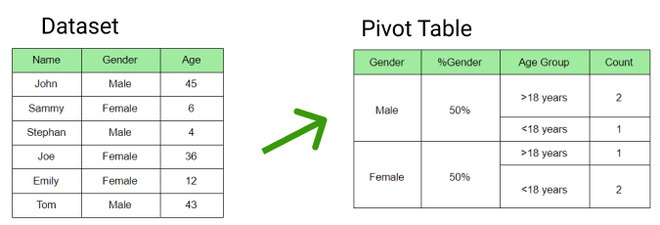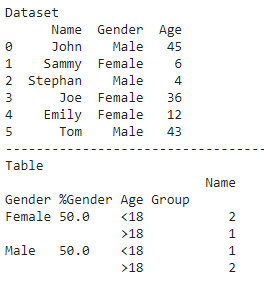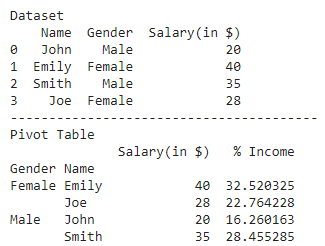# How to include percentage in pivot table in Pandas?

• Last Updated : 24 Jan, 2021

Seaborn is an amazing visualization library for statistical graphics plotting in Python. It provides beautiful default styles and color palettes to make statistical plots more attractive. It is built on the top of matplotlib library and also closely integrated into the data structures from pandas.

Pivot table is used to summarize data which includes various statistical concepts. To calculate the percentage of a category in a pivot table we calculate the ratio of category count to the total count. Below are some examples which depict how to include percentage in a pivot table:

Attention geek! Strengthen your foundations with the Python Programming Foundation Course and learn the basics.

To begin with, your interview preparations Enhance your Data Structures concepts with the Python DS Course. And to begin with your Machine Learning Journey, join the Machine Learning - Basic Level Course

Example 1:

In the figure below, the pivot table has been created for the given dataset where the gender percentage has been calculated.## Python3

 `# importing pandas library``import` `pandas as pd`` ` `# creating dataframe``df ``=` `pd.DataFrame({``'Name'``: [``'John'``, ``'Sammy'``, ``'Stephan'``, ``'Joe'``, ``'Emily'``, ``'Tom'``],``                   ``'Gender'``: [``'Male'``, ``'Female'``, ``'Male'``,``                              ``'Female'``, ``'Female'``, ``'Male'``],``                   ``'Age'``: [``45``, ``6``, ``4``, ``36``, ``12``, ``43``]})``print``(``"Dataset"``)``print``(df)``print``(``"-"``*``40``)`` ` `# categorizing in age groups``def` `age_bucket(age):``    ``if` `age <``=` `18``:``        ``return` `"<18"``    ``else``:``        ``return` `">18"`` ` `df[``'Age Group'``] ``=` `df[``'Age'``].``apply``(age_bucket)`` ` `# calculating gender percentage``gender ``=` `pd.DataFrame(df.Gender.value_counts(normalize``=``True``)``*``100``).reset_index()``gender.columns ``=` `[``'Gender'``, ``'%Gender'``]``df ``=` `pd.merge(left``=``df, right``=``gender, how``=``'inner'``, on``=``[``'Gender'``])`` ` `# creating pivot table``table ``=` `pd.pivot_table(df, index``=``[``'Gender'``, ``'%Gender'``, ``'Age Group'``], ``                       ``values``=``[``'Name'``], aggfunc``=``{``'Name'``: ``'count'``,})`` ` `# display table``print``(``"Table"``)``print``(table)`

Output:Example 2:

Here is another example which depicts how to calculate the percentage of a variable to its sum total in a particular column:

## Python3

 `# importing required libraries``import` `pandas as pd``import` `matplotlib.pyplot as plt`` ` `# creating dataframe``df ``=` `pd.DataFrame({``    ``'Name'``: [``'John'``, ``'Emily'``, ``'Smith'``, ``'Joe'``],``    ``'Gender'``: [``'Male'``, ``'Female'``, ``'Male'``, ``'Female'``],``    ``'Salary(in \$)'``: [``20``, ``40``, ``35``, ``28``]})`` ` `print``(``"Dataset"``)``print``(df)``print``(``"-"``*``40``)`` ` `# creating pivot table``table ``=` `pd.pivot_table(df, index``=``[``'Gender'``, ``'Name'``])`` ` `# calculating percentage``table[``'% Income'``] ``=` `(table[``'Salary(in \$)'``]``/``table[``'Salary(in \$)'``].``sum``())``*``100`` ` `# display table``print``(``"Pivot Table"``)``print``(table)`

Output:My Personal Notes arrow_drop_up